{itemname} {itemname}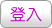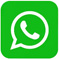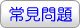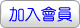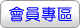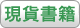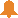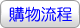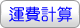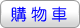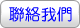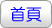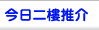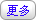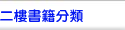Calculus & Its Applications （GE）（14版）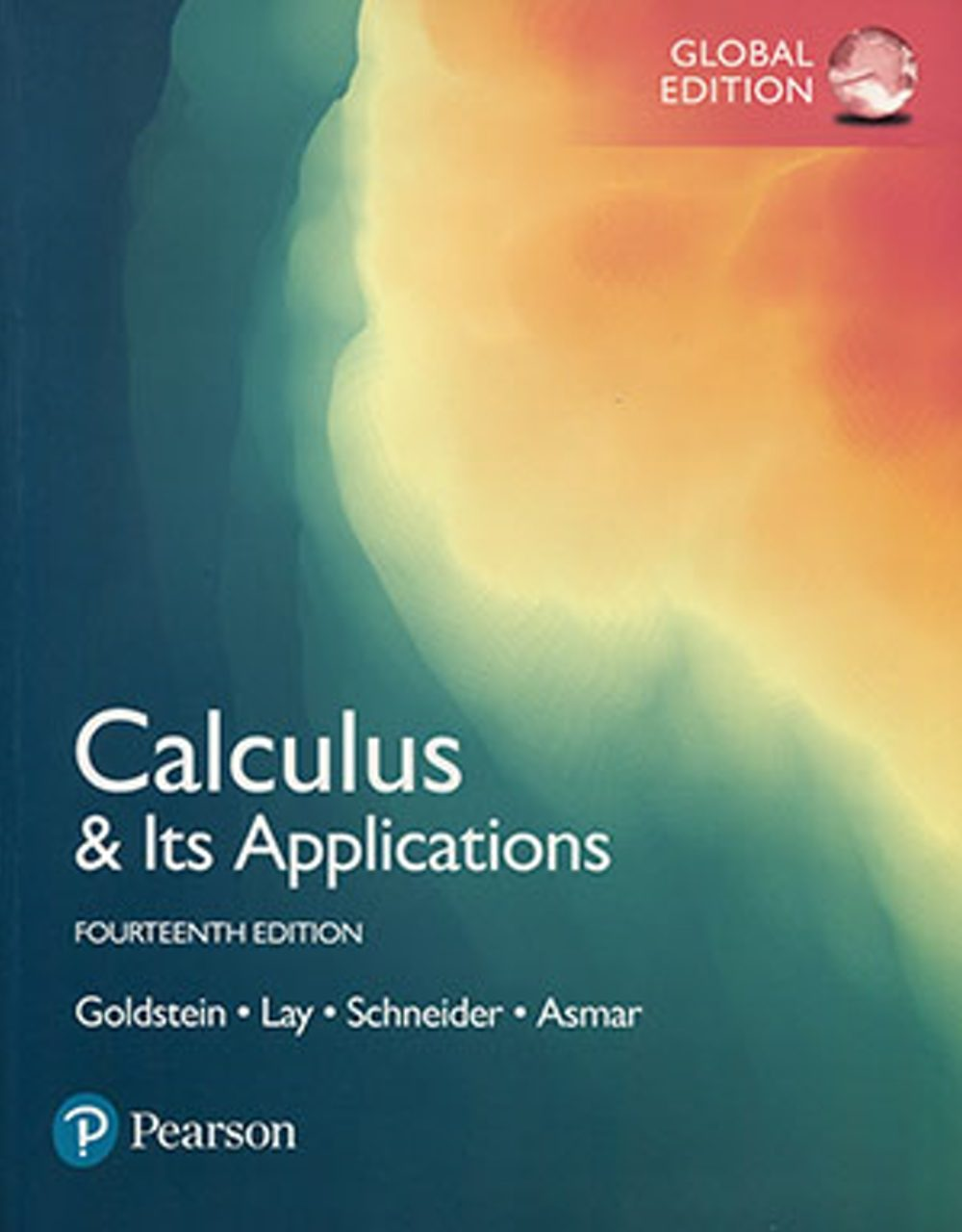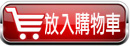沒有庫存 訂購需時10-14天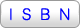9781292229041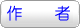Larry J. Goldstein,David C. Lay,David I. Schneider,Nakhle H. Asmar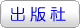華泰文化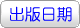2018年9月05日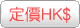460.00  元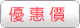HK\$ 437省下 \$23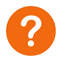ISBN：9781292229041
• 叢書系列：微積分
• 規格：平裝 / 610頁 / 21.7 x 27.7 x 2 cm / 普通級 / 全彩印刷 / 14版
• 出版地：台灣
微積分

• 專業/教科書/政府出版品 > 數理化類 > 數學

Calculus & Its Applications builds intuition with key concepts of calculus before the analytical material. For example, the authors explain the derivative geometrically before they present limits, and they introduce the definite integral intuitively via the notion of net change before they discuss Riemann sums.

The strategic organisation of topics makes it easy to adjust the level of theoretical material covered. The significant applications introduced early in the course serve to motivate students and make the mathematics more accessible. Another unique aspect of the text is its intuitive use of differential equations to model a variety of phenomena in Chapter 5, which addresses applications of exponential and logarithmic functions.

Ch 0 Functions

Ch 1 The Derivative

Ch 2 Applications of the Derivative

Ch 3 Techniques of Differentiation

Ch 4 The Exponential and Natural Logarithm Functions

Ch 5 Applications of the Exponential and Natural Logarithm Functions

Ch 6 The Definite Integral

Ch 7 Functions of Several Variables

Ch 8 The Trigonometric Functions

Ch 9 Techniques of Integration

Ch10 Differential Equations

Ch11 Taylor Polynomials and Infinite Series

Ch12 Probability and Calculus

 其 他 著 作